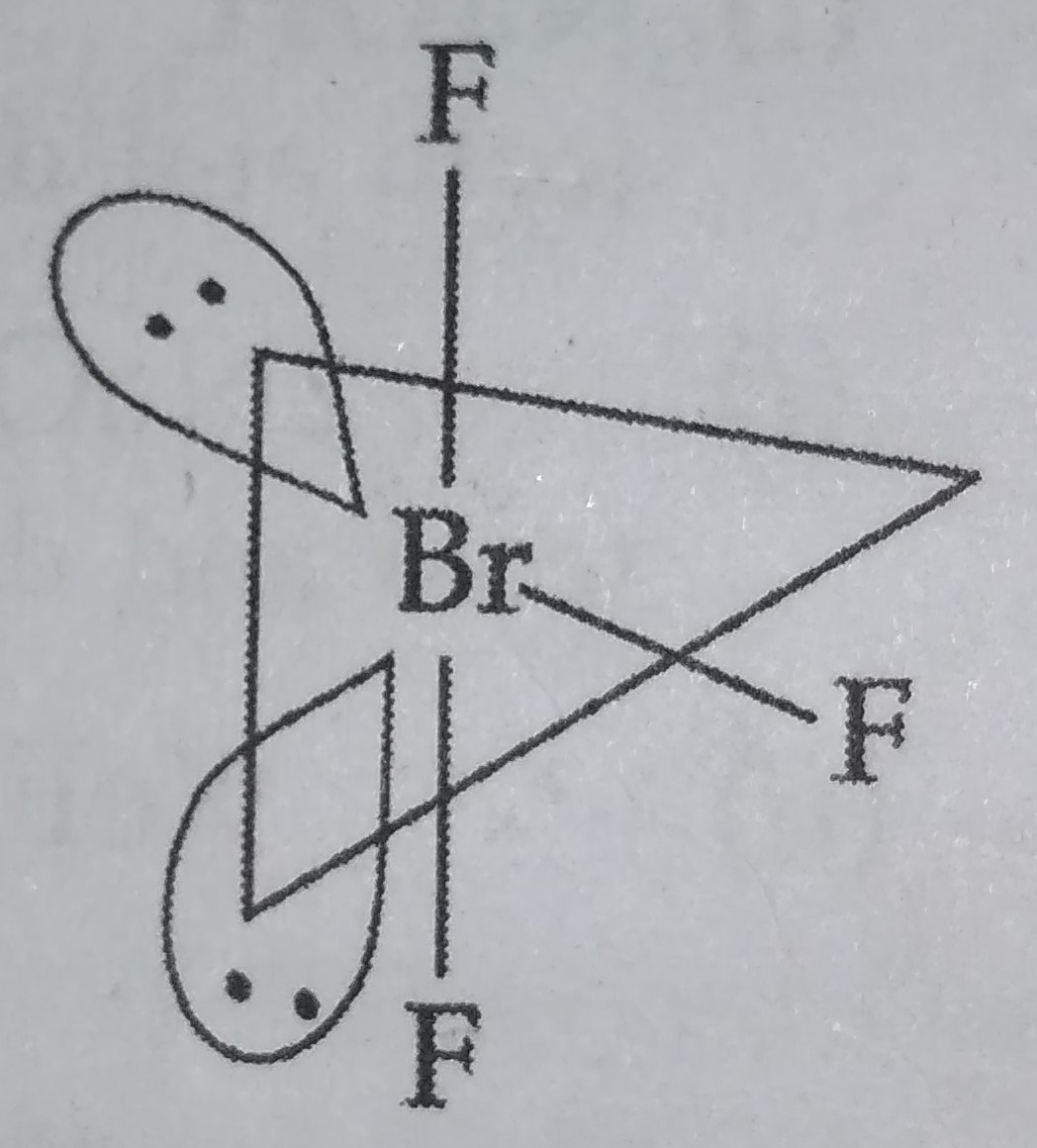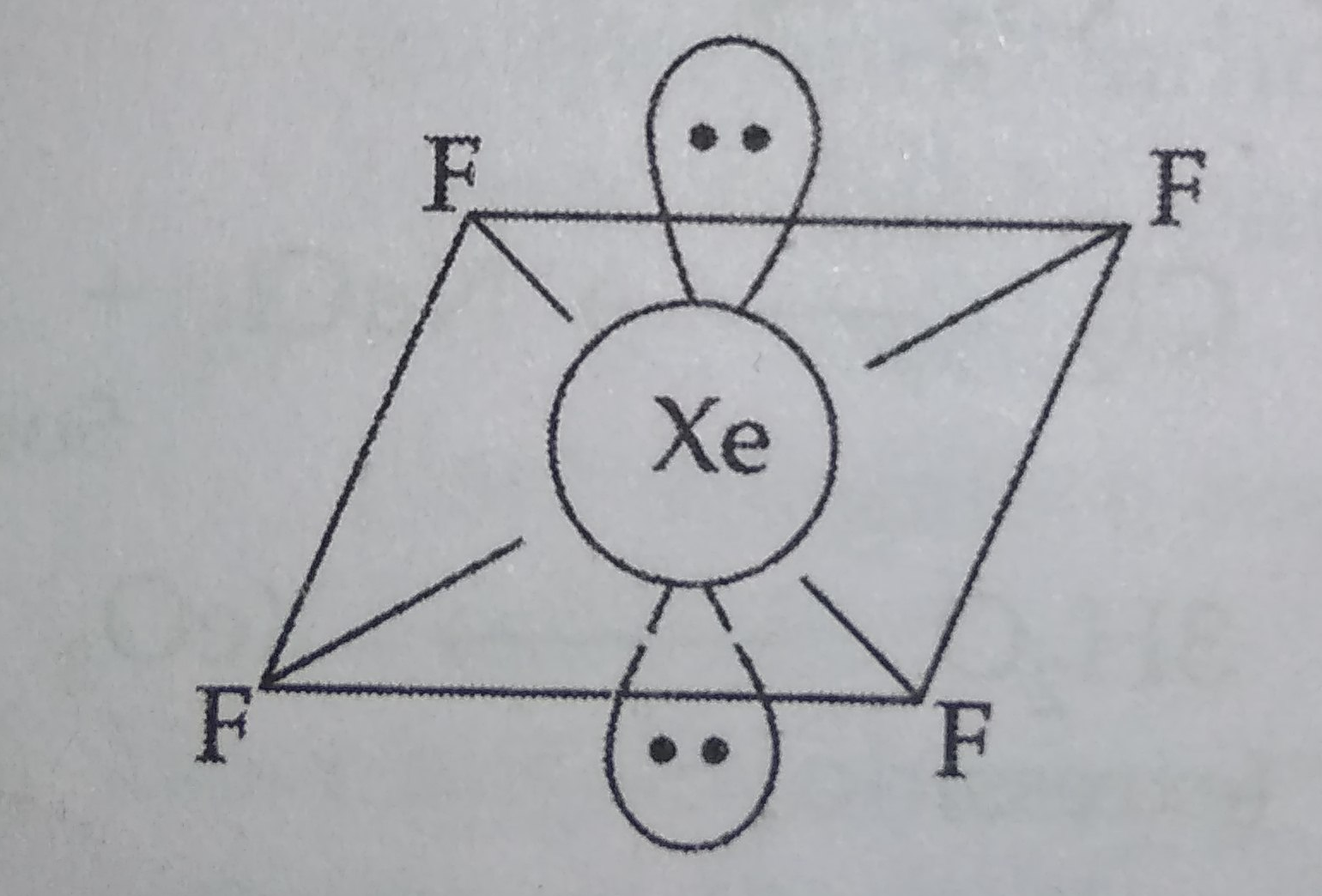Draw the structural formulae of molecules of following compounds.

(i) $Br{F}_{3}$ and                                  (ii) $Xe{F}_{4}$

Concept Videos :-

#8 | 18th Group

Concept Questions :-

group 18,perparation and properties

(i) $Br{F}_{3}$Shape : T-shape

(ii) $Xe{F}_{4}$Shape : Square planar

Difficulty Level:

• 82%
• 0%